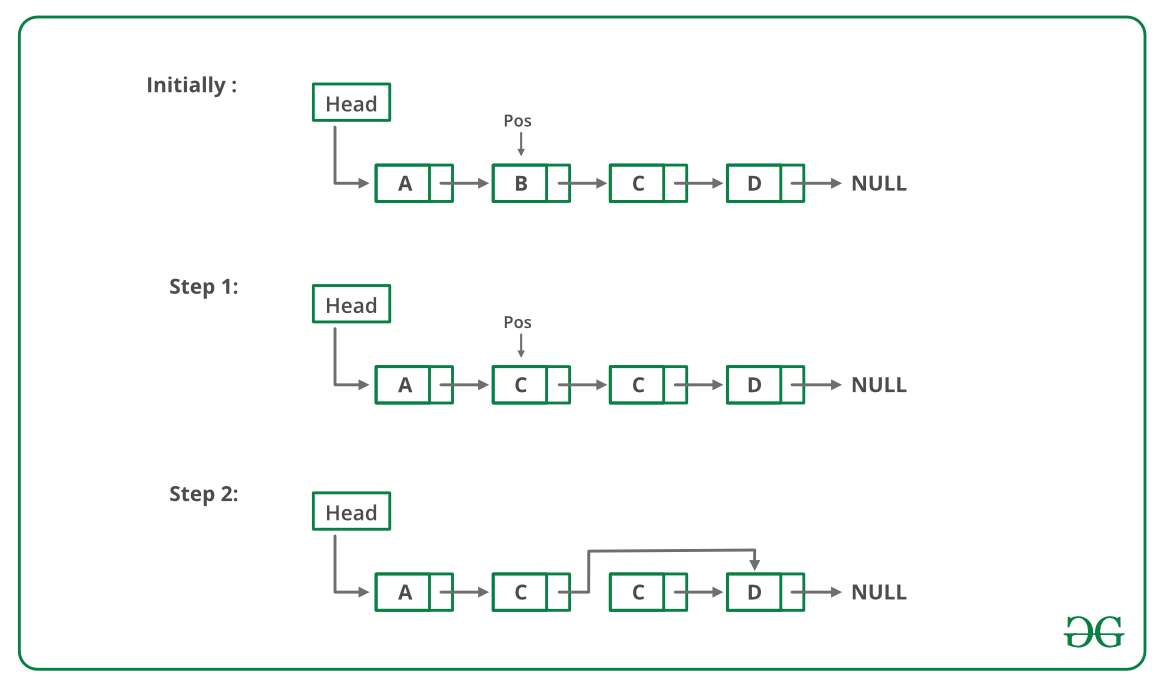• Difficulty Level : Easy
• Last Updated : 24 Jun, 2022

You are given a singly linked list and pointer which is pointing to the node which is required to be deleted. Any information about head pointer or any other node is not given. You need to write a function to delete that node from linked list. Your function will take only one argument, i.e., pointer to the node which is to be deleted.

Note: No head reference is given to you. It is guaranteed that the node to be deleted is not the last node.

A linked list is built as:Definition of each node is as follows:
struct Node {
int data;
struct Node* next;
};

Examples:

Consider below LL for given examples:Input : C (a pointer to C)

Output : A–>B–>D–>E–>F

Input : A (a pointer to A)

Output : B–>D–>E–>F

Approach:

Why conventional deletion method would fail here?
It would be a simple deletion problem from the singly linked list if the head pointer was given because for deletion you must know the previous node and you can easily reach there by traversing from the head pointer. Conventional deletion is impossible without knowledge of the previous node of a node that needs to be deleted.

How to delete the node when you dont have the head pointer?
The trick here is we can copy the data of the next node to the data field of the current node to be deleted. Then we can move one step forward. Now our next has become the current node and the current has become the previous node. Now we can easily delete the current node by conventional deletion methods.

Illustration:

For example, suppose we need to delete B and a pointer to B is given.

If we had a pointer to A, we could have deleted B easily.
But here we will copy the data field of C to the data field of B. Then we will move forward.

Now we are at C and we have a pointer to B i.e. the previous pointer. So we will delete C.

That’s how node B will be deleted.

Below image is a dry run of the above approach:Below is the implementation of the above approach :

## CPP

 `// C++ program to delete a node``#include ``using` `namespace` `std;`` ` `/* Link list node */``struct` `Node {``    ``int` `data;``    ``struct` `Node* next;``};`` ` `// Function to delete the node without head``void` `deleteNodeWithoutHead(``struct` `Node* pos)``{``    ``if` `(pos == NULL) ``// If linked list is empty``        ``return``;``    ``else` `{`` ` `        ``if` `(pos->next == NULL) {``            ``printf``(``"This is last node, require head, can't "``                   ``"be freed\n"``);``            ``return``;``        ``}`` ` `        ``struct` `Node* temp = pos->next;`` ` `        ``// Copy data of the next node to current node``        ``pos->data = pos->next->data;`` ` `        ``// Perform conventional deletion``        ``pos->next = pos->next->next;`` ` `        ``free``(temp);``    ``}``}`` ` `// Function to print the linked list``void` `print(Node* head)``{``    ``Node* temp = head;``    ``while` `(temp) {``        ``cout << temp->data << ``" -> "``;``        ``temp = temp->next;``    ``}`` ` `    ``cout << ``"NULL"``;``}`` ` `void` `push(``struct` `Node** head_ref, ``int` `new_data)``{``    ``/* allocate node */``    ``struct` `Node* new_node = ``new` `Node();`` ` `    ``/* put in the data */``    ``new_node->data = new_data;`` ` `    ``/* link the old list off the new node */``    ``new_node->next = (*head_ref);`` ` `    ``/* move the head to point to the new node */``    ``(*head_ref) = new_node;``}`` ` `// Driver Code``int` `main()``{``    ``/* Start with the empty list */``    ``struct` `Node* head = NULL;`` ` `    ``// create linked 35->15->4->20``    ``push(&head, 20);``    ``push(&head, 4);``    ``push(&head, 15);``    ``push(&head, 35);``    ``cout << ``"Initial Linked List: \n"``;``    ``print(head);``    ``cout << endl << endl;`` ` `    ``// Delete 15 without sending head``    ``Node* del = head->next;``    ``deleteNodeWithoutHead(del);`` ` `    ``// Print the final linked list``    ``cout << ``"Final Linked List after deletion of 15:\n"``;``    ``print(head);`` ` `    ``return` `0;`` ` `    ``// This code has been contributed by Striver``}`

Output

```Initial Linked List:
35 -> 15 -> 4 -> 20 -> NULL

Final Linked List after deletion of 15:
35 -> 4 -> 20 -> NULL```